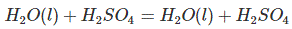# MCQs on Electric Circuits

##### Page 34 of 63. Go to page 1 2 3 4 5 6 7 8 9 10 11 12 13 14 15 16 17 18 19 20 21 22 23 24 25 26 27 28 29 30 31 32 33 34 35 36 37 38 39 40 41 42 43 44 45 46 47 48 49 50 51 52 53 54 55 56 57 58 59 60 61 62 63
01․ Cells are connected in series in order to
increase the voltage rating.
increase the current rating.
increase the life of cells.
none of above.

When connecting the cells in series, according to the Kirchhoff’s law the voltage across the cells can be increased and at the same time, the current capacity of the cells is the same as the capacity of the single cell.

02․ Common impurity in battery electrolyte is
sodium chloride.
iron.
dust.

The iron content in the electrolyte leads to make a conductive path from positive plate to negative plate. That is nothing but short circuit occurs in between the positive and negative plate. Hence, iron is considered as the common impurity in the electrolyte.

03․ The indication of the state of charge of a battery is best given by
specific gravity of electrolyte.
temperature of electrolyte.
colour of electrolyte.
level of electrolyte.

During the charging and discharging time, the value of specific gravity of electrolyte varies. For example, during the charging time, the value of specific gravity increases from the current level and during the discharging time, the value of specific gravity decreases from the current level. But, the rest of all factors which shown in above are not changed. Hence, the state of charge of a battery is given by the specific gravity of electrolytic.

04․ One ampere hour charge is equivalent to
36 coulombs.
360 coulombs.
3600 coulombs.
36000 coulombs.

1 Ampere-hour = (1 Coulomb/Second) × 3600 Seconds = 3600 Coulombs. This is the reason that the one ampere-hour charge is equivalent to 3600 Coulombs.

05․ The internal resistance of a dry cell is of the order of
0.2 to 0.4 Ohm.
1 to 1.5 Ohm.
2 to 5 Ohm.
1 to 15 Ohm.

In dry-cell battery , the electrolyte is in the form of a paste. And there is no presence of nickel and iron material. Due to that, the internal resistance of the dry cell is very less. The value is approximately in between 0.2 to 0.4Ω.

06․ When water is added to sulphuric acid
colour of acid changes.
lot of heat is generated.
water start boiling.
acid particles get separated.

Due to the exothermic reaction, the heat is generated when the sulphuric acid is added with water. The expression is as follows,The amount of heat (q) in Joules can be calculated as follows: q = specific heat of water X grams of water X change in temperature Specific heat of water = 4.18 J / (g °C) Grams of water = 50 Change in temperature = final temperature - initial temperature

07․ A constant voltage source has
low internal resistance.
high internal resistance.
minimum efficiency.
minimum current capacity.

If the internal voltage is too less, then the voltage drop across the terminal is also less. Hence, we can get the constant voltage. In this condition, the source voltage is almost equal to the terminal voltage.

08․ Which battery is used in aeroplanes?
Dry cell battery.
Edission cell.
None of them.

In aero planes, mostly we are using the nickel - cadmium battery. So, none of them is correct answer.

09․ The current in a chemical cell is a movement of
positive hole charges.
positive ions only.
negative ions only.
positive and negative ions.

We know that the Ions with a positive charge are called positive ions and ions with a negative charge are called negatives. These two ions move from an area of high energy toward an area of low energy that produces a flow of electrons (current). Basically, this movement of ions is called as electrolytic conduction.

10․ Cells are connected in parallel to
increase the voltage output.
increase the internal resistance.
decrease the current capacity.
increase the currentcapacity.

As we know that if battery are connected in parallel then the voltage across the terminal is constant. But, all battery deliver their own current capacity in that single terminal. Hence, finally the current capacity of the whole system increases.

<<<3233343536>>>